## Example Questions

← Previous 1 3 4 5 6 7 8 9

### Example Question #1 : How To Evaluate Algebraic Expressions

Quantitative Comparison

0 < x < 1

A

---

(2x + 5)/(x2)

B

---

5x

Quantity B is greater

The relationship cannot be determined from the information given

Quantity A is greater

The two quantities are equal

Quantity A is greater

Explanation:

Since A is a fraction with an exponential term in the denominator, its maximum value is when x is at a minimum. In B, the maximum value is when x approaches its maximum. Therefore, we can check whether there is overlap between the two quantities: No matter how close to either 0 or 1 x reaches, A will always be greater than B. (In fact, the minimum value for A is ~7, while the maximum value of B is ~5)

Be sure to keep your value of x consistent when plugging between the two fractions! The question asks for when they have the same x-value, not for when they are solved independently.

### Example Question #2 : How To Evaluate Algebraic Expressions

If x = -4 and y = 7, what is the value of 3x-5y?

-45
-47
47
23
-23
Explanation:

Substitute the values into equation:  3(-4) - 5(7) = -12 - 35 = -47.

### Example Question #3 : How To Evaluate Algebraic Expressions

Quantitative Comparison

Quantity A: x

Quantity B: 2x

Quantity A is greater.

Quantity B is greater.

The relationship cannot be determined from the information given.

The two quantities are equal.

The relationship cannot be determined from the information given.

Explanation:

For a quantitative comparison question such as this one, it is best to first plug in the numbers 0, 1, and –1. Plugging in 0 gets the same answer for both columns. Plugging in 1 makes Quantity B bigger. Plugging in –1 makes Quantity A bigger. Therefore the answer cannot be determined.

### Example Question #261 : Algebra

A store sells 17 coffee mugs for $169. Some of the mugs are$12 each and some are $7 each. How many$7 coffee mugs were sold?

9

10

8

6

7

7

Explanation:

Write two independent equations that represent the problem.

x + y = 17 and 12x + 7y = 169

If we solve the first equation for x, we get x = 17 – y and we can plug this into the second equation.

12(17 – y) + 7y = 169

204 – 12y + 7y =169

–5y = –35

y = 7

Kim has 22 coins made up of quarters, nickles, and dimes that total $2.45. Kim has twice as many nickles as quarters. How many dimes does she have? Possible Answers: 7 10 Cannot be determined 12 5 Correct answer: 7 Explanation: The answer is 7. Let us first write down three equations that represent the problem: n + d + q = 22 2q = n 5n + 10d + 25q = 245 Lets plug the second equation into the first and third equations: (2q) + d + q = 22 5(2q) + 10d + 25q = 245 Solve the first equation for d and plug into the last equation: d = 22 – 3q 10q + 10(22 – 3q) + 25q = 245 Solve for q. 220 – 30q + 25q + 10q = 245 5q = 25 q = 5 Therefore, n = 10 and d = 7 ### Example Question #2 : Evaluating Expressions In the equation ax + b = 32, x is a constant. If a = 3 when b = 2, what is a when b = 12? Possible Answers: 2 10 7 3 13 Correct answer: 2 Explanation: The answer is 2. First solve for the constant x: 3x + 2 = 32 x = 10 Now plug in x = 10 and b = 12: a(10) + 12 = 32 a = 2 ### Example Question #6 : How To Evaluate Algebraic Expressions A specialty socket wrench, typically priced at$29.99, is on sale for 30% off. An additional 45% is discounted at the register. What is the final sale price of the wrench?

$4.95$4.05

$7.50$11.55

$22.49 Correct answer:$11.55

Explanation:

The answer is $11.55 The original cost is$29.99 but we are going to discount 30%, meaning we will only pay 70%. The new prices is 29.99 x 0.70 = $20.99. The new price is then dicounted an additional 45%, meaning we will only pay 55% of the new price. The final price is 20.99 x 0.55 =$11.55.

### Example Question #7 : How To Evaluate Algebraic Expressions

Quantitative Comparison

x is an integer.

Quantity A: (x + 1)2

Quantity B: (x – 1)2

Quantity A is greater.

Quantity B is greater.

The relationship cannot be determined from the information given.

The two quantities are equal.

The relationship cannot be determined from the information given.

Explanation:

When picking numbers, we should always try to plug in the numbers 0, 1, and –1 first.

First try 0:

(0 + 1)2 = 1

(0 – 1)2 = 1

Here the two quantities are equal.

Now try 1:

(1 + 1)2 = 4

(1 – 1)2 = 0.

Here Quantity A is greater.

Therefore the relationship cannot be determined.

### Example Question #8 : How To Evaluate Algebraic Expressions

Barry's workout today consists of 10 squats every minute on the minute and 6 situps every other minute for 1 hour.  How many squats and situps does Barry do in total?

1200

800

960

1000

780

780

Explanation:

squats: 10 squats * 60 minutes = 600 squats

situps: 6 situps * 30 minutes = 180 situps

total = 600 + 180 = 780

### Example Question #1 : Evaluating Expressions

Letand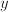be integers such that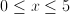and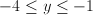.

Quantity A                    Quantity B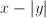0

Quantity B is greater

The relationship cannot be determined from the information given

Quantity A is greater

Quantity A and Quantity B are equal

The relationship cannot be determined from the information given

Explanation:

The quantityproduces a minimum of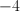and a maximum of 4, which are less than and greater than 0, respectively. Therefore, the answer cannot be determined from the information given.

← Previous 1 3 4 5 6 7 8 9

Tired of practice problems?

Try live online GRE prep today.